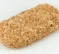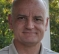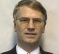## What is the maximum output amplitude of an IIR filter?Started by 3 years ago35 replieslatest reply 3 years ago859 views

When implementing a fixed-point digital filter, I need to think about overflow/saturation.

For a FIR filter, the maximum +/- output values (OUTPUT+ and OUTPUT-) are easy to define in terms of the input extremes INPUT+ and INPUT-. For example, if the inputs are 16-bit signed twos compliment, then INPUT+ = 32767 and INPUT- = -32768, and:

• OUTPUT+: Occurs when all positive-valued taps are fed with INPUT+ and all negative-valued taps are fed with INPUT-.
• OUTPUT-: Vice versa.

If we approximate 32767 ≈ 32768, then the output extremes are approximately sum(abs(taps)) times larger than the input extremes. This can mean, for example, that even a filter that attenuates all frequencies (magnitude response <0dB everywhere) can produce time-domain output samples larger than any input sample.

However, I don't know how to approach IIR filters. Of course, I don't care about cases where the output can explode without bound. But for "well-behaved" (stable) IIR filters, is there a way to calculate this, or some sensible approximation?

[ - ]You need to control the gain of your IIR to check maximum dynamic range at output.

For example the leaky integrator:

y = a.x(n) + (1-a).y(n-1)

gives unity dc gain if a < 1 otherwise it could build up for ever.

[ - ]@kaz As I said in my original post, even if gain (in the frequency domain) is < 0dB across all frequencies, the output amplitude (in the time domain) can still be greater than the largest possible input amplitude.

Therefore, showing that gain at DC (or any other frequency) is bounded to 0 dB doesn't seem to tell me how many bits are required to represent the largest possible output value.

For example, any FIR filter's DC gain is sum(taps), but its maximum amplitude growth is approximately sum(abs(taps)). So any FIR filter with both positive and negative taps will have greater amplitude growth than its DC gain. In this sense (for FIR filters), the DC gain is some sort of lower bound, but I am looking for the upper bound (for IIR filters).

[ - ]I believe that the size of the internal variables inside the fixed point IIR filters is actually the harder problem to deal with.
Most IIR filters are > order than the simple leaky integrator.

My rule of thumb is that the growth of the internal variables is proportional to the inverse of the distance from the poles to the unit circle.

So, if the poles have magnitude of 0.9, then the gain of the internal variables will be at 10x the input level, or more.

These internal variables are quite likely to bite if you are not careful.

I often allow them to be carried at 32 bits, even if all the data are within a 16 bit input and output word.

I realize that this was not necessarily your question, but just thought that I would comment.

David

[ - ]@dgshaw6 In my current work, I am only dealing with 1st order filters. Does your rule of thumb apply there too?

With all of the test signals I have tried, I haven't seen any growth greater than a factor of 2 (in fact, I don't think I have seen > 1.5). This is assuming the filter is always normalized to have maximum 0dB gain in the frequency domain.

Is there perhaps a better test signal I should be using to produce larger growth? (I have tried chirps, sinewaves, and Gaussian and uniform noise).

[ - ]In the work that I was doing, the problem showed up in very narrow bandpass filters, which are almost always either a single biquad or combination of biquads.

I have to confess, that I have not experimented with this theory in the first order case.  However, intuition suggests that 2X input might be the upper limit, as you suggest.

Another thought suddenly comes to mind.  What happens with a step function as the input?
It seems possible, that the fact that a first order filter, has by definition, a pole in-line with DC, that DC would be the signal that would most likely trigger the issue.

[ - ]I suppose you could generate an impulse response for your IIR and do the same thing.

[ - ]@dszabo An impulse will not typically produce the largest possible amplitude at the output of the filter (in the time domain).

[ - ]Say you used that impulse response as an FIR filter, would you know what to do then

[ - ]@dszabo Ah, now I see what you're saying! Great idea. I'll give it a try.

[ - ]@dszabo This worked really well (once I remembered to flip the order of the FIR taps to get the convolution in the correct order).

I wasn't really sure how to choose the number of FIR taps, so I just kept increasing until the results looked stable. (In my case, a couple of million taps seems to be more than plenty).

In the worst case, one of my filters shows amplitude growth of 2.02, which is almost double what any of my other test signals produced.

This gives me confidence that I can just add 2 bits and never need to worry about it again.

Thanks very much for your idea!

[ - ]Recently I read the following article, fixed-point-iir-filter-challenges, which suggests to generate the impulse response and:

"You can assume that the system under study is asymptotically stable. This assumption ensures that the impulse-response vector, h[m], essentially converges to zero by a finite-sample index m≤M. Because M is finite, you can compute the impulse response at each shift register and attendant L1 -norm Gmax:i in finite time."

So it seems you are on the right track.

[ - ]@hirnprinz Thank you very much for sharing that article. That was exactly the track I ended up going down. After reading the article, it seems so obvious, but I was really scratching my head for a while!

I'll need to sift through the section on quantization error, as I think I could benefit equally from that. I spent many hours trawling through simulation results, when I think a couple of sums on the back of a postcard could perhaps have done the trick.

[ - ]How can we represent an IIR filter as "really" equivalent FIR using the IIR impulse response no matter how long is FIR model. Doesn't IIR mean infinite and has its own inner structure for computation? so each internal node has history back infinitely which is only limited by memory precision at that node

[ - ]Honestly?  It was kind of a guess on my part that this would even work. But I figured it’s not like the internals are some random crazy thing, it’s just a delay line. So if I know that the input and output are bounded, the internals must be as well.

[ - ]@kaz As the name suggests, an exact representation would typically need infinitely many FIR taps (in the same way that we would need an infinite sample rate and infinitely many bits in our ADC to exactly digitize an analog signal).

However, as long as the filter is asymptotically stable (which in many applications - including mine - it is required to be), you can get get as close as you want to exactness by increasing the number of FIR taps.

In my case, 1 or 2 million FIR taps were easily enough to have converged to more decimal places than I could be bothered to write down.

[ - ]If you apply dc input to FIR with same sign as coeffs you get maximum possible output, I am trying to prove that impulse input can't even get FIR max output right.

Impulse is ok for checking frequency response, not max output

%%% input giving max output %%%

h = fir1(50,.2);

x = sign(h).*ones(1,length(h));

y = filter(h,1,x);

max(y)/max(x)

%%% input as impulse %%%

x = zeros(1,length(h));

x(1) = 1;

y = filter(h,1,x);

max(y)/max(x)

[ - ]@kaz For a FIR filter, the impulse response (y) is the filter (h). At the end of your code, if you compare y and h, you will find they are identical.

So you have missed a step. Before calculating the final max(y)/max(x), you need to apply the filter. You can do that by inserting these two lines:

x = sign(h);
y = filter(y,1,x);


Of course, this will give exactly the same result as using h directly.

[ - ]My test is about what input leads to max output. Let me recode:

filter has dc power unity as its sum is 1

%%%%%%%%%%%%%%%%%%%%%%%%%%%%%%%%

h = fir1(50,.2);

x1 = [1 zeros(1,100)];          %impulse

x2 = ones(1,length(h));         %dc

x3 = sign(h).*ones(1,length(h));%dc with coeff polarity

x4 = h;                         %input as coeffs

y1 = filter(h,1,x1);

y2 = filter(h,1,x2);

y3 = filter(h,1,x3);

y4 = filter(h,1,x4);

[max(y1)/max(x1),max(y2)/max(x2),max(y3)/max(x3),max(y4)/max(x4)]

[ - ]@kaz If you don't impose any constraints on the input signal, then the output signal can be infinitely large (e.g. if you multiply all of your input signals by Inf).

Therefore, it makes sense to constrain all of your test inputs in the same way to produce fair results. In particular, x4 should be h/max(abs(h)) so that its maximum amplitude is 1, like all the others.

As I wrote in my original post, the theoretical maximum output is produced using x = flip(sign(h)). Anything else will produce magnitudes less than or equal to that (as long as the input amplitudes are constrained fairly).

[ - ]That brings us to the issue of normalisation/scaling. Let us forget x4 as I just added it as example input. If you do FPGA as opposed to software then you can see code below scaled/quantised exactly as in hardware:

%%%%%%%%%%%%%%%%%%%%%%%%%%%

h = fir1(50,.2);

h = round(2^15*h);

x1 = 2^15*[1 zeros(1,100)];          %impulse

x2 = 2^15*ones(1,length(h));         %dc

x3 = 2^15*sign(h).*ones(1,length(h));%dc with coeff polarity

y1 = round(filter(h,1,x1)/2^15);

y2 = round(filter(h,1,x2)/2^15);

y3 = round(filter(h,1,x3)/2^15);

[max(x1),max(x2),max(x3)]

[max(y1),max(y2),max(y3)]

%%%%%%%%%%%%%%%%%%%%%%%%%%%

so x1,x2,x3 max is 32768 but y3 is maximum possible

[ - ]@kaz Quantization doesn't change anything.

We can constrain the maximum input amplitude to be any number (quantized or not - it doesn't make any difference).

And rounding the outputs can't suddenly make a smaller number somehow overtake a larger number (at least not with any sane rounding algorithm).

[ - ]Sorry we are not in sync now...I am proving my point that for same bitwidth FIR filter (input/output) the maximum output is case y3, not y1,y2. Good luck

[ - ]The Wikipedia 'Stability' section https://en.wikipedia.org/wiki/Infinite_impulse_res... maybe a useful place to start.

Separating the variables will be part of the analysis, which will depend on how the IIR is formulated.

[ - ]@philipoakley Maybe I can understand better using a specific example. I have a filter with this transfer function:

$$H(z) = \frac{1 - z^{-1}}{1 - Az^{-1}}$$

where A depends on the configuration, but is always < 1. For example, a typical value may be A = 0.98.

Therefore, if I understand correctly, this filter is guaranteed to be stable because poles exist where z = A (and A is always inside the unit circle).

However, I can't see how to connect this to the maximum output amplitude of the filter. Maybe it helps to write out the time-domain impulse response and look at that?

[ - ]so, I think you have:

y[n] = 0.98*y[n-1] + (x[n] - x[n-1])

Assuming that y[-1] = 0, you have a 2% decay on any existing y[n-1] value, plus the difference between the current and previous x values.

If x[-1] = 0, you should find that there is no way to exceed the maximum output level as there is no growing integral value. The maximum initial difference would be +/-INPUT, and after that 2*INPUT, but the previous y[] would be lagging and of the opposite sign, so no overflow.

That said, it is just a few coefficient tweaks away of being unstable. It's always good engineering to include a suitable limiter if such changes are possible.

[ - ]@philipoakley Thank you. This sounds logical. I need to mull it over, but I think this is going in the right direction...

[ - ]In the below filter model you can experiment.

But it will be sensitive to internal multiplication/rounding which I haven't modelled.

You will see input is power all over and output max can exceed input max.

%%%%%%%%%%%%%%%%%%%%%%%%%%%%%%%%%%%%%%%%

x = randn(1,2^16);

x = round(2^10*x);

y(1) = x(1);

for n = 2:length(x)

y(n) = 0.98*y(n-1) + (x(n) - x(n-1));

end

max(y)/max(x)

[ - ]@kaz I have written fixed-point software models that include the internal rounding.

I have tested with a wide variety of input signals (including Gaussian noise, like in your example).

Depending on the input signal (and the filter configuration), I can measure different maximum output values.

However, I can only test with a finite number of test signals and I don't have any reason to believe that any of my test signals cover the "worst case". (Certainly, none of them represent a worst case for a FIR filter).

So my question is about how to cover the worst case (not just the worst test signal I tried).

[ - ]Practically I would lower input max and/or add clipping logic to output.

Finding out the worst input pattern for a given input/IIR filter is no trivial task and can make a good research task I believe.

The primary target of design is to keeping healthy dynamic range and clip that occasional output.

[ - ]Yes, this is basically what I am researching.

If we reduce the input amplitude, then the question remains: "by how much should it be reduced (for a given filter)?".

If we implement clipping, then the question remains: "is clipping more efficient than adding the optimal number of bits required to guarantee clipping is avoided?"

Both these questions could be answered if I could determine the maximum possible output amplitude (and internal amplitudes, for more complicated filters).

[ - ]The maximum value is determined by the initial y {aka y(1)} value copied from x {x(1)}, so the randomised trial will get rather random results because the maximum y value is all decided by that first dice roll value.

Try initialising the settings with  x(1) = 0; y(1) = 0; to see the impact.

[ - ]An IIR filter output can be larger than the input, like with an analog filter by the way. Everything depends on the gain you apply for fo (also called resonance or emphasis)

In music applications, there are many LPF based on IIR cells (not all of them however). These filters must be followed by a clipping stage to make sure the filter remains stable (especially if the filter is implemented in fixed point format)

So everything depends on the coefficients and the filter model you want. Did you take a look to Robert Brisow Jonhson work? He has transposed all 2nd order cells into digital domain, and the formulas he has written down show the resonance factor very easily

[ - ]In a basic sense it's a design decision that you have control over.   You control the gain of the filter, and you control the sizes of the registers used and therefore the output precision.   As mentioned by BEBSynthesizers, you need to put a clipper on the output to prevent wraparound at your chosen output precision.

[ - ]